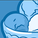# New to Qlik Sense

If you’re new to Qlik Sense, start with this Discussion Board and get up-to-speed quickly.

Announcements
cancel
Showing results for
Did you mean:Creator III

## Table with sum and percentage

I am having some issues with a table that will tell me the number of errors and the percentage all in one field. I was able to do this for a separate table, but since this table is set up a little different, I am having some issues.

I am trying to show the last few days of data as a dimension and separated by area.My expression for the measure is as followed (side note: the first if statement I tested Area 8, showing true to the above image, first step was to test the sum of all Area's, then from there I would add the division to get actual percentage, if there are any tips or any other ways to do this, please help, Thanks):

sum(Errors_DOC_RV)

&

' Errors / '

&

if(Area_DOC_RV = 'Area 8',

(

(Sum({\$<Area_DOC_RV = {"Area 1"} >}Errors_DOC_RV))

+

(Sum({\$<Area_DOC_RV = {"Area 2"} >}Errors_DOC_RV))

+

(Sum({\$<Area_DOC_RV = {"Area 3"} >}Errors_DOC_RV))

+

(Sum({\$<Area_DOC_RV = {"Area 4"} >}Errors_DOC_RV))

+

(Sum({\$<Area_DOC_RV = {"Area 5"} >}Errors_DOC_RV))

+

(Sum({\$<Area_DOC_RV = {"Area 6"} >}Errors_DOC_RV))

+

(Sum({\$<Area_DOC_RV = {"Area 7"} >}Errors_DOC_RV))

+

(Sum({\$<Area_DOC_RV = {"Area 8"} >}Errors_DOC_RV))

+

(Sum({\$<Area_DOC_RV = {"Area 9"} >}Errors_DOC_RV))

),

if( Area_DOC_RV = 'Decon', sum(Inc_Data_DOC_RV),

if(Area_DOC_RV = 'Area 2', sum(Miss_Paper_DOC_RV),

if(Area_DOC_RV = 'Area 3', sum(Write_illeg_DOC_RV),

if(Area_DOC_RV = 'Area 4', sum(Other_DOC_RV),

if(Area_DOC_RV = 'Area 5', sum(Other_DOC_RV),

if(Area_DOC_RV = 'Area 6', sum(Other_DOC_RV),

if(Area_DOC_RV = 'Area 7', sum(Other_DOC_RV),

if(Area_DOC_RV = 'Area 1', sum(Other_DOC_RV),

if(Area_DOC_RV = 'Area 9', sum(Other_DOC_RV)

))))))))))

&

'%'

2 RepliesCreator III

Hi,

could you share some data? I am wondering why are you using such complicated expression. Could you a little bit explain what does or should does your calculation?Creator III
Author

Attached is a sample file with the same issue. Based on the data, the result for 9/3/2018 - area1, should read " 5 errors / 16%", 9/4/2018 - area1, should read "7 errors / 17%", and 9/5/2018 - area1 should read "4 errors / 18%"

Again, I'm sure there's a lot easier way of doing this, i'm just stuck with this one.

This is my expression:

sum(errors)

&

' Errors / '

&

if(area = 'area1',

(

(Sum({\$<area = {"area1"} >}errors))

+

(Sum({\$<area = {"area2"} >}errors))

+

(Sum({\$<area = {"area3"} >}errors))

+

(Sum({\$<area = {"area4"} >}errors))

+

(Sum({\$<area = {"area5"} >}errors))

)

,

IF(area = 'area2', Sum(errors),

if( area = 'area3', sum(errors),

if( area = 'area4', sum(errors),

if(area = 'area5', sum(errors)

)))))

&

'%'